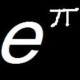## Using calculus to generate the quadratic formula

by

I get (unreasonable considerable) joy in finding fancy new proofs of elementary results, proofs that might come under the heading of mathematics made difficult or obscure. (I’ve never seen Linderholm’s book, but suspect it would appeal to a twisted part of my psyche.) I don’t quite understand the psychology behind this liking, but there it is nonetheless.

One of my favorite examples follows: solving quadratic equations using integration to complete the square.

Suppose$f(x)=ax^2+bx+c$, and we want to find the solutions to$f(x)=0$.

Note that$f'(x) = 2ax+b$ and$f(0) = c$, and thus it must be that$f(x) = c+ \int_0^x 2at+b \; dt$.

We compute this antiderivative using the change of variables$w=2at+b, \quad dw = 2a\;dt$, which leads to$c + \int_b^{2x+b} \frac1{2a} \; w \; dw$. This last expression is equal to$c + \left( \frac1{4a} (2ax+b)^2 - \frac{b^2}{4a} \right)$.

Thus the roots of$f(x) = 0$ are found by solving the equation$c + \frac{ (2ax+b)^2 - b^2 }{4a} = 0$.

This leads to$(2ax+b)^2 -b^2 = -4ac$, and thus$2ax+b = \pm \sqrt{b^2 - 4ac}$, and so finally we arrive at the roots$x = \frac{ -b \pm \sqrt{b^2 - 4ac}}{2a}$.

Forthcoming: Using Fubini’s Theorem to prove Integration by Parts

### 6 Responses to “Using calculus to generate the quadratic formula”

1.Integration by parts via iterated integrals « 360 Says:

[…] of an occasional series, featuring obfuscated proofs of elementary results.  Previously: Using calculus to generate the quadratic formula.  […]

2. 42nd Carnival of Mathematics — The Endeavour Says:

[…] at the 360 blog presents two fun articles using advanced techniques to prove elementary results, Using calculus to derive the quadratic equation and Integration by parts via iterated […]

3.trevorpythag Says:

much more interesting than the “completing the sqaure” proof we learnt in school!!

4. AP Calculus AB: Tuesday, 2/17/2009 « Dr. Cooper’s Wyoming Seminary Math Weblog Says:

[…] Challenge problem: Derive the quadratic formula using integration. The solution is here. […]

5.C.B.Kay Says:

An alternative ‘proof’ for the solution to the roots of the quadratic equation. I begin with differentiation.

(1) y = ax*x + bx + c

dy/dx = 2ax + b = 0 at a turning point.

x = -b/2a at turning point

quadratic roots are some distance ‘s’ each side of the t.pt.

(2) i.e. -b/2a (+or-) s

Substituting into (1) with y=0 for the roots, gives:-

0 = a(-b/2a + s)*(-b/2a + s) + b(-b/2a + s) + c

reversing inside the brackets gives:-

0 = a(s – b/2a)*(s – b/2a) + b(s – b/2a) + c

0 = a(s*s – bs/a + b*b/4a*a) + bs – b*b/2a + c

0 = as*s – bs + b*b/4a + bs – b*b/2a + c

-bs cancels with +bs giving:-

0 = as*s + b*b/4a – b*b/2a + c

b*b/4a – b*b/2a = -b*b/4a giving:-

0 = as*s -b*b/4a + c

Multiplying across by 4a gives:-

0 = 4a*a*s*s – b*b + 4ac

4a*a*s*s = b*b – 4ac

2as = sqrt( b*b – 4ac)

s = (sqrt(b*b-4ac))/2a

From (2) roots are:-

(-b +or- sqrt(b*b-4ac))/2a

I would welcome feedback. I have never seen this as a proof before.

6. 42nd Carnival of Mathematics Says:

[…] at the 360 blog presents two fun articles using advanced techniques to prove elementary results, Using calculus to derive the quadratic equation and Integration by parts via iterated […]